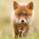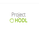3674 visitas
3674
EXPERIMENTAL:Testing the strategy tester :D
```//@version=2
strategy("[STRATEGY][RS]MA Strategy test V0", overlay=true, pyramiding=3, default_qty_type=strategy.percent_of_equity, default_qty_value=1.0, initial_capital=100000)
length0 = input(5)
length1 = input(21)

isinsession = not na(time('1', '0400-1500'))
price = open

ma0 = ema(ema(price, length0), length0)
ma1 = ema(ema(price, length1), length1)
plot(ma0, color=navy)
plot(ma1, color=black)

osc0 = price-ma0
osc1 = ma0-ma1

isbull = osc0 > 0 and osc1 > 0
buy_condition = isinsession and isbull and not isbull
buy_exit_condition = osc0 < 0 and osc1 < 0

isbear = osc0 < 0 and osc1 < 0
sell_condition = isinsession and isbear and not isbear
sell_exit_condition = osc0 > 0 and osc1 > 0
strategy.entry("sell", strategy.short, comment="sell", when=sell_condition)
strategy.close(id='sell', when=sell_exit_condition)

//plot(strategy.equity, title="equity", color=red, linewidth=2, style=areabr)```